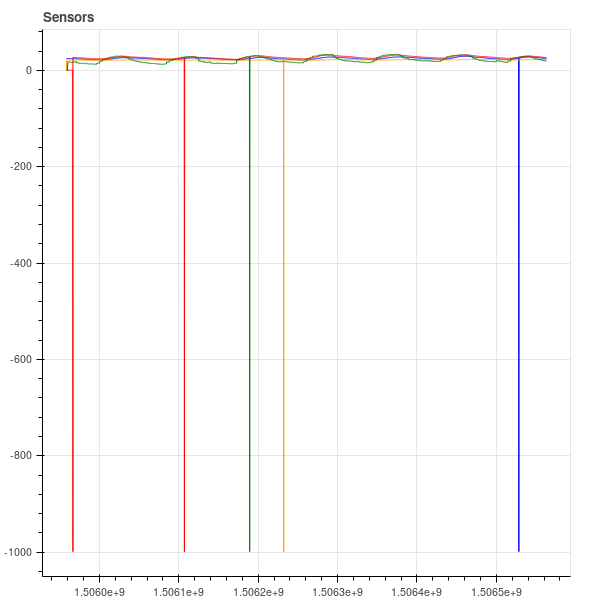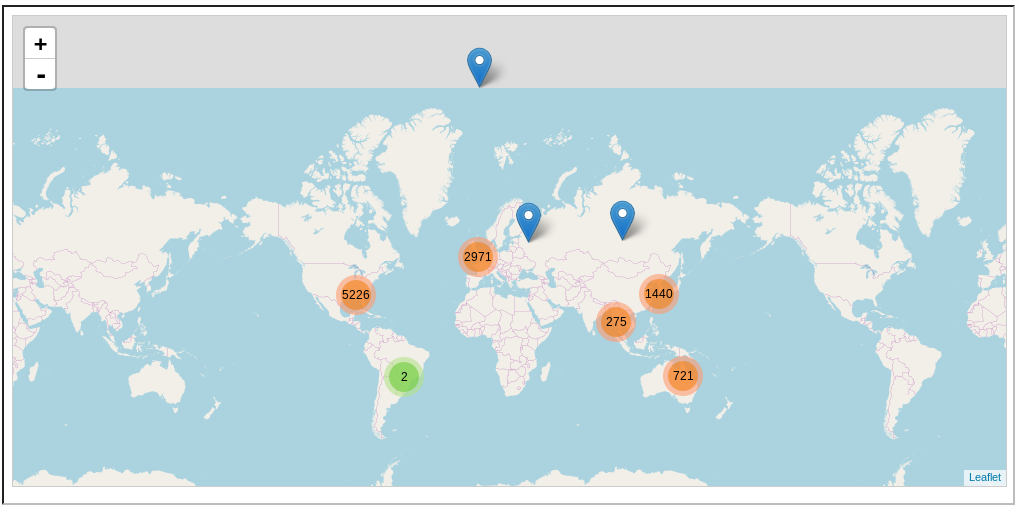CSE 662 Fall 2019 - Dynamic Imputation

November 25

## Missing Data

18-34
35-49
50-64
65+

### Errors### ErrorsProblem: You want to analyze a new dataset where specific values are missing or clearly wrong.

### Drop Invalid Data

SELECT * FROM R WHERE X IS NOT NULL

Easy and fast.
Compatible with scale-free aggregates (max, min, avg).
Breaks scaling aggregates (sum, count).
Can be unsafe if data is correlated.

### Interpolation

$X_i = \begin{cases} X_i &\textbf{if } \exists X_i\\ \frac{X_{i+1}+X_{i-1}}{2}& \textbf{otherwise}\end{cases}$

Can be fast.
Rarely a problem with correlations.
Requires an appropriate order...
...if one even exists

### "Hot Deck" Imputation

Replace invalid values with the last valid value

Usually Accurate.
General.
Requires shuffling and/or sorting.

### Mean Value Imputation

Replace invalid values with the column mean

Easy and somewhat fast.
General.
Requires computing the mean.

### Classifier Imputation

Replace invalid values with the column mean

General.
Resilient to correlations.
Requires training a classifier.

### Missing Values

Drop Missing Values
Fast
X Imputation
Accurate

Which to use?

### Observations

• Some columns are not relevant to the current workload.
• Some rows will get filtered out by query operators.
• Joins might fill in missing correlations.

Idea: Make the query optimizer aware of imputation needs.

### In-Engine Imputation

Two new operators enforce COLUMN NOT NULL constraints.

Drop ($\delta_{C}$)
Non-blocking operator discards tuples if $C = \texttt{NULL}$.
Impute ($\mu_{C}$)
Blocking operator that replaces $C$ when $C = \texttt{NULL}$.
Train + Repair in one step.

### Challenges

1. $\delta$ or $\mu$?
2. Where in the plan to repair?

Basic query optimization problem!

The user manually identifies "dirty" input table columns.

Intuition: An attribute is dirty if it contains nulls that must be repaired.

Constraint: A dirty attribute must be repaired before it is used (e.g., by a selection or aggregate).

Question: Which columns are dirty?

$$\textbf{Dirty}(R) = \text{The dirty columns of R}$$

$$\textbf{Dirty}(\pi_C(q)) = \textbf{Dirty}(q) \cap C$$

$$\textbf{Dirty}(\sigma(q)) = \textbf{Dirty}(q)$$

$$\textbf{Dirty}(q_1 \bowtie q_2) = \textbf{Dirty}(q_1) \cup \textbf{Dirty}(q_2)$$

$$\textbf{Dirty}(\delta_C(q)) = \textbf{Dirty}(\mu_C(q)) = \textbf{Dirty}(q) - C$$

Cost Model

Original Query → Optimizer → Optimized Query

### A Classical Query Optimizer

• Selection Pushdown
• Join Reordering
• Join Selection
• Projection Inlining
• ... and more ...

### Optimization Rules

When a query subtree matches a specific pattern...

... (optionally) replace it with a different pattern.

### Imputation

Question: How to inject repair operators?

Challenge: Changes require re-evaluating the cost model.

Idea: Restrict variations in columns being repaired.

### Imputation

Before each operator...

• Repair only dirty columns used by the operator.
OR
Repair all dirty columns in the input.
• Drop OR Impute

($2\times 2 = 4$ options for each operator)

Optimize the query normally.

Try every plan that satisfies the constraint on dirty columns.

Optimize for precision and time.

### Accelerating the Search

• Cache performance/cardinality/dirty column results for intermediate subtrees
• Drop/ignore subtrees with the same dirty column set that are worse in both time and precision than another plan.

### Cost Model

Need to predict the computational cost of the plan.

Need to predict the accuracy of the result.

For both, we need histograms.

### Histograms

Propagating per-column histograms is a standard part of query optimizers

How do $\delta$ and $\mu$ propagate histograms.

1. Figure out the number of rows (cardinality) after the operator.
2. Rescale the histograms to the new number of rows.

### Drop ($\delta_C$)

Conservative assumption of full overlap between NULLs

1. $\textbf{card}(\delta_C(R)) = \textbf{card}(R) \cdot \max_{col}(R.col.\text{hist}[\texttt{NULL}])$
2. $\delta_C(R).col.\text{hist}[v] = R.col.\text{hist}[v]$ (except $v = \texttt{NULL}$)

### Impute ($\mu_C$)

Assume distribution of values unchanged

1. $\textbf{card}(\mu_C(R)) = \textbf{card}(R)$
2. $\mu_C(R).col.\text{hist}[v] = R.col.\text{hist}[v] \cdot \frac{\textbf{card}(R)}{\textbf{card}(R) - R.col.\text{hist}[\texttt{NULL}]}$ (except $v = \texttt{NULL}$)

### Estimating Precision

Given: Penalty function $P(\mu_C(q)) \in [0,1]$

0 = perfect replacement

1 = as bad as dropping all columns in $C$

### Example: Decision Trees

$$|C|\cdot \frac{1}{\sqrt{|\textbf{attrs}(q)|\cdot \textbf{card}(q)}}$$

### Query Penalty

$$L_P(q) = \begin{cases} 1 + L(q') & \textbf{if } q = \delta_C(q') \\ P(q) + L(q') & \textbf{if } q = \mu_C(q') \\ L(q_1) + L(q_2) & \textbf{if } q = q_1 \bowtie q_2\\ L(q') & \textbf{if } q = \sigma(q') \textbf{ or } q = \pi(q')\\ 0 & \textbf{if } q \text{ is a table} \end{cases}$$

Total penalties judged relative to a plan that drops all attributes.

### Estimated Query Cost

Handled as normal by the query optimizer.

• $\delta_C \approx \sigma$
• $\mu_C$ cost estimated by given function $T(q)$.

### Concerns

Later imputation means more opportunities to drop tuples naturally, but some joins produce larger output relations.

Larger data is sometimes better (more opportunities to find correlations), but not always.

### Concerns

Penalty function is measured per-operator rather than per-column.